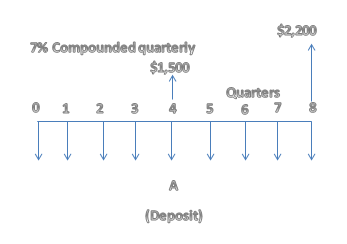# What is the amount of the quarterly deposits A such that you will be able to withdraw the amounts...

## Question:

What is the amount of the quarterly deposits A such that you will be able to withdraw the amounts shown in the cash flow diagram if the interest rate is 7% compounded quarterly?The amount of the quarterly deposits A should be _____.

## Present Value of Annuity:

A stream of cash flows which are equal in magnitude and are equally spaced over time is called as annuity. If the annuity is paid or received at the end of every period, it is called as ordinary annuity and if the annuity is paid or received at the beginning of every period, it is called as annuity due.

The calculated amount of the quarterly deposits A is $359.02. The quarterly interest rate is given by: • = Annual interest rate / number of quarters in a year • {eq}= \dfrac{7\%}{4} {/eq} • = 1.75% The value of the quarterly deposits (A) can be figured out by equating the sum of the present value of the quarterly deposits with the sum of the present value of all the withdrawals given in the cash flow diagram at a quarterly interest rate of 1.75% (or 0.0175) as shown below: {eq}\dfrac{\$1,500}{1.0175^4} + \dfrac{\$1,500}{1.0175^8} = \dfrac{A}{1.0175^0} + \dfrac{A}{1.0175^1} + ....... + \dfrac{A}{1.0175^8} {/eq} On solving the above equation we get, annual quarterly deposits (A) =$359.02